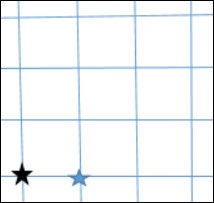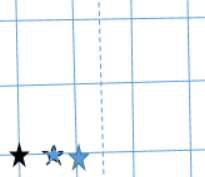# Redshift Vs. Kinematic Doppler Shift

A galaxy which is at redshift z = 10, corresponds to v≈80% of c. The mass of the Milky Way is around 1011M⊙, if we consider the dark matter, it is 1012M⊙. Our milky way is thus massive. If it moves at 80% of c, it does not fit in the general concept of how objects move.

We know,

$$\frac{v_r}{c} = \frac{\lambda_{obs} - \lambda{rest}}{\lambda_{rest}}$$

For small values of z,

$$z = \frac{v_r}{c} = \frac{\lambda_{obs}-\lambda_{rest}}{\lambda_{rest}}$$

In the following graph, the class between flux and wavelength, there are emission lines on top of the continuum. From the H-α line information, we get to conclude that roughly z = 7. This implies galaxy is moving at 70% of c. We are observing a shift and interpreting it as velocity. We should get rid of this notion and look at z in a different way. Imagine space as a 2D grid representing the universe as shown below.Consider the black star to be our own milky way and the blue star to be some other galaxy. When we record light from this galaxy, we see the spectrum and find out its redshift i.e., the galaxy is moving away. When the photon was emitted, it had relative velocity.

• What if the space was expanding?

• It is an instantaneous redshift of photon. Cumulative redshifts along the space between two galaxies will tend to a large redshift. The wavelength will change finally. It is the expansion of the space rather than the kinematic movement of the galaxies.

The following image shows if mutual gravity overflows expansion then this is not participating in the Hubble’s law.In the Kinematic Doppler Shift, the redshift is induced in a photon at the time of emission. In a Cosmological Redshift, in every step, it is getting cumulatively redshifted. In a gravitational potential, a photon will get blue shifted. As it crawls out of gravitational potential, it gets redshifted.

As per a Special Theory of Relativity, two objects passing by each other cannot have a relative velocity greater than the speed of light. The velocity we speak about is of the expansion of the universe. For large values of z, the redshift is cosmological and not a valid measure of the actual recessional velocity of the object with respect to us.

## The Cosmological Principle

It stems from the Copernicus Notion of the universe. As per this notion, the universe is homogeneous and isotropic. There is no preferred direction and location in the universe.

• Homogeneity means no matter which part of the universe you reside in, you will see universe is the same in all parts. Isotropic nature means no matter which direction you look, you are going to see the same structure.

• A fitting example of homogeneity is a Paddy field. It looks homogeneous from all parts, but when wind flows, there are variations in its orientation, thus it is not isotropic. Consider a mountain on a flat land and an observer is standing on the mountain top. He will see the isotropic nature of the flat land, but it is not homogeneous. If in a homogeneous universe, it is isotropic at a point, it is isotropic everywhere.

• There have been large scale surveys to map the universe. Sloan Digital Sky Survey is one such survey, which did not focus much on the declination, but on the right ascension. The lookback time is around 2 billion years. Every pixel corresponds to the location of a galaxy and the color corresponds to the morphological structure. The green color represented blue spiral galaxy while the red false color indicated massive galaxies.

• Galaxies are there in a filamentary structure in a cosmological web and there are voids in between the galaxies.

• $\delta M/M \cong 1$ i.e., fluctuation of mass distribution is 1 M is the mass of the matter present within a given cube. In this case, take the volume 50 Mpc cube.

• For a cube side of 1000 Mpc, $\delta M/M \cong 10^{−4}$.

• One way to quantify homogeneity is to take mass fluctuations. Mass fluctuations will be higher at lower scales.

• For quantifying the isotropic nature, consider cosmic microwave background radiation. Universe is nearly isotropic at large angular scales.

### Points to Remember

• Two objects passing by each other cannot have a relative velocity greater than the speed of light.

• Cosmological Principle states that the universe is homogeneous and isotropic.

• This homogeneity exists at a very large angular scale and not on smaller scales.

• SDSS (Sloan Digital Sky Survey) is an effort to map the night sky, verifying the Cosmological Principle.